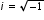# Cluster 1: Perform arithmetic operations with complex numbers. (Algebra 2 - Additional Cluster)ArchivedExport Print

Clusters should not be sorted from Major to Supporting and then taught in that order. To do so would strip the coherence of the mathematical ideas and miss the opportunity to enhance the major work of the grade with the supporting clusters.

General Information
Number: MAFS.912.N-CN.1
Title: Perform arithmetic operations with complex numbers. (Algebra 2 - Additional Cluster)
Type: Cluster
Subject: Mathematics - Archived
Domain-Subdomain: Number & Quantity: The Complex Number System

## Related Standards

This cluster includes the following benchmarks.

## Related Access Points

This cluster includes the following access points.

## Access Points

MAFS.912.N-CN.1.AP.1a
Identify i as the square root of -1.
MAFS.912.N-CN.1.AP.1b
Identify a number in the form a + bi as a complex number.
MAFS.912.N-CN.1.AP.2a
Use properties to combine like terms of complex numbers in the form of a + bi with a and b being real numbers.
MAFS.912.N-CN.1.AP.2b
Use properties to multiply complex numbers in the form of a + bi with a and b being real numbers.

## Related Resources

Vetted resources educators can use to teach the concepts and skills in this topic.

## Lesson Plans

This lesson unit is intended to help you assess how well students are able to:

• Use the properties of exponents, including rational exponents, and manipulate algebraic statements involving radicals.
• Discriminate between equations and identities.

There is also an opportunity to consider the role of the imaginary number, but this is optional.

Type: Lesson Plan

Can You Hear Me Now? Using Cell Phone Signals to Divide Complex Numbers:

Students always ask, “What’s the point of complex numbers?” One application of complex numbers is cell phone signals and their wavelengths. This lesson introduces division of complex numbers by hooking students in with the topic of cell phones. Students will attempt to calculate a cell phone signal wavelength involving complex numbers and discover the use of complex conjugates. Then, students will practice with complex number division using a Kagan strategy called Rally Coach.  To conclude, students will use white boards and dry erase markers to review the concepts covered in class.

Type: Lesson Plan

Computations with Complex Numbers:

This resource involves simplifying algebraic expressions that involve complex numbers and various algebraic operations.

## Tutorials

Multiplying Complex Numbers:

This video demonstrates how to multiply complex numbers using distributive property and FOIL method.

Type: Tutorial

How to Subtract Complex Numbers:

This video will demonstrate how to subtract complex numbers.

Type: Tutorial

This video will demonstrate how to add complex numbers.

Type: Tutorial

Introduction to i and imaginary numbers:

This video gives an introduction to 'i' and imaginary numbers. From this tutorial, students will learn the rules of imaginary numbers.

Type: Tutorial

## Video/Audio/Animation

MIT BLOSSOMS - Fabulous Fractals and Difference Equations :

This learning video introduces students to the world of Fractal Geometry through the use of difference equations. As a prerequisite to this lesson, students would need two years of high school algebra (comfort with single variable equations) and motivation to learn basic complex arithmetic. Ms. Zager has included a complete introductory tutorial on complex arithmetic with homework assignments downloadable here. Also downloadable are some supplemental challenge problems. Time required to complete the core lesson is approximately one hour, and materials needed include a blackboard/whiteboard as well as space for students to work in small groups. During the in-class portions of this interactive lesson, students will brainstorm on the outcome of the chaos game and practice calculating trajectories of difference equations.

Type: Video/Audio/Animation

## Student Resources

Vetted resources students can use to learn the concepts and skills in this topic.

Computations with Complex Numbers:

This resource involves simplifying algebraic expressions that involve complex numbers and various algebraic operations.

## Tutorials

Multiplying Complex Numbers:

This video demonstrates how to multiply complex numbers using distributive property and FOIL method.

Type: Tutorial

How to Subtract Complex Numbers:

This video will demonstrate how to subtract complex numbers.

Type: Tutorial

This video will demonstrate how to add complex numbers.

Type: Tutorial

Introduction to i and imaginary numbers:

This video gives an introduction to 'i' and imaginary numbers. From this tutorial, students will learn the rules of imaginary numbers.

Type: Tutorial

## Video/Audio/Animation

MIT BLOSSOMS - Fabulous Fractals and Difference Equations :

This learning video introduces students to the world of Fractal Geometry through the use of difference equations. As a prerequisite to this lesson, students would need two years of high school algebra (comfort with single variable equations) and motivation to learn basic complex arithmetic. Ms. Zager has included a complete introductory tutorial on complex arithmetic with homework assignments downloadable here. Also downloadable are some supplemental challenge problems. Time required to complete the core lesson is approximately one hour, and materials needed include a blackboard/whiteboard as well as space for students to work in small groups. During the in-class portions of this interactive lesson, students will brainstorm on the outcome of the chaos game and practice calculating trajectories of difference equations.

Type: Video/Audio/Animation

## Parent Resources

Vetted resources caregivers can use to help students learn the concepts and skills in this topic.